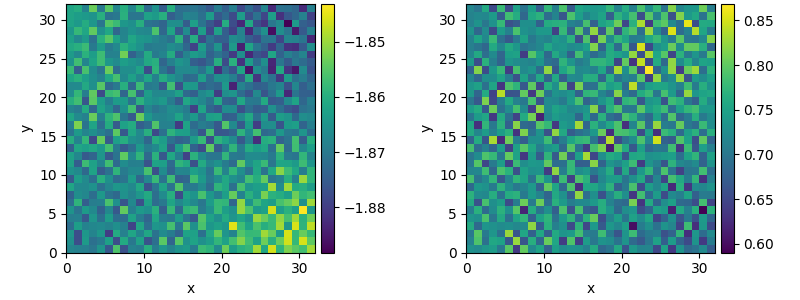# 2.20. Custom Class for coupled PDEs

This example shows how to solve a set of coupled PDEs, the spatially coupled FitzHugh–Nagumo model, which is a simple model for the excitable dynamics of coupled Neurons:

$\begin{split}\partial_t u &= \nabla^2 u + u (u - \alpha) (1 - u) + w \\ \partial_t w &= \epsilon u\end{split}$

Here, $$\alpha$$ denotes the external stimulus and $$\epsilon$$ defines the recovery time scale. We implement this as a custom PDE class below.0%|          | 0/100.0 [00:00<?, ?it/s]
Initializing:   0%|          | 0/100.0 [00:00<?, ?it/s]
0%|          | 0/100.0 [00:00<?, ?it/s]
0%|          | 0.24/100.0 [00:00<01:36,  1.03it/s]
1%|          | 0.72/100.0 [00:00<00:38,  2.58it/s]
3%|▎         | 2.93/100.0 [00:00<00:15,  6.09it/s]
8%|▊         | 7.88/100.0 [00:00<00:10,  8.47it/s]
15%|█▌        | 15.25/100.0 [00:01<00:09,  8.97it/s]
24%|██▎       | 23.65/100.0 [00:02<00:08,  9.14it/s]
33%|███▎      | 32.57/100.0 [00:03<00:07,  9.57it/s]
42%|████▏     | 42.46/100.0 [00:04<00:05,  9.73it/s]
53%|█████▎    | 52.53/100.0 [00:05<00:04,  9.66it/s]
62%|██████▏   | 62.26/100.0 [00:06<00:03,  9.86it/s]
73%|███████▎  | 72.66/100.0 [00:07<00:02,  9.88it/s]
83%|████████▎ | 82.84/100.0 [00:08<00:01,  9.86it/s]
93%|█████████▎| 92.79/100.0 [00:09<00:00,  9.97it/s]
93%|█████████▎| 92.79/100.0 [00:10<00:00,  9.25it/s]
100%|██████████| 100.0/100.0 [00:10<00:00,  9.97it/s]
100%|██████████| 100.0/100.0 [00:10<00:00,  9.97it/s]


from pde import FieldCollection, PDEBase, UnitGrid

class FitzhughNagumoPDE(PDEBase):
"""FitzHugh–Nagumo model with diffusive coupling"""

def __init__(self, stimulus=0.5, τ=10, a=0, b=0, bc="auto_periodic_neumann"):
super().__init__()
self.bc = bc
self.stimulus = stimulus
self.τ = τ
self.a = a
self.b = b

def evolution_rate(self, state, t=0):
v, w = state  # membrane potential and recovery variable

v_t = v.laplace(bc=self.bc) + v - v**3 / 3 - w + self.stimulus
w_t = (v + self.a - self.b * w) / self.τ

return FieldCollection([v_t, w_t])

grid = UnitGrid([32, 32])
state = FieldCollection.scalar_random_uniform(2, grid)

eq = FitzhughNagumoPDE()
result = eq.solve(state, t_range=100, dt=0.01)
result.plot()


Total running time of the script: (0 minutes 10.482 seconds)